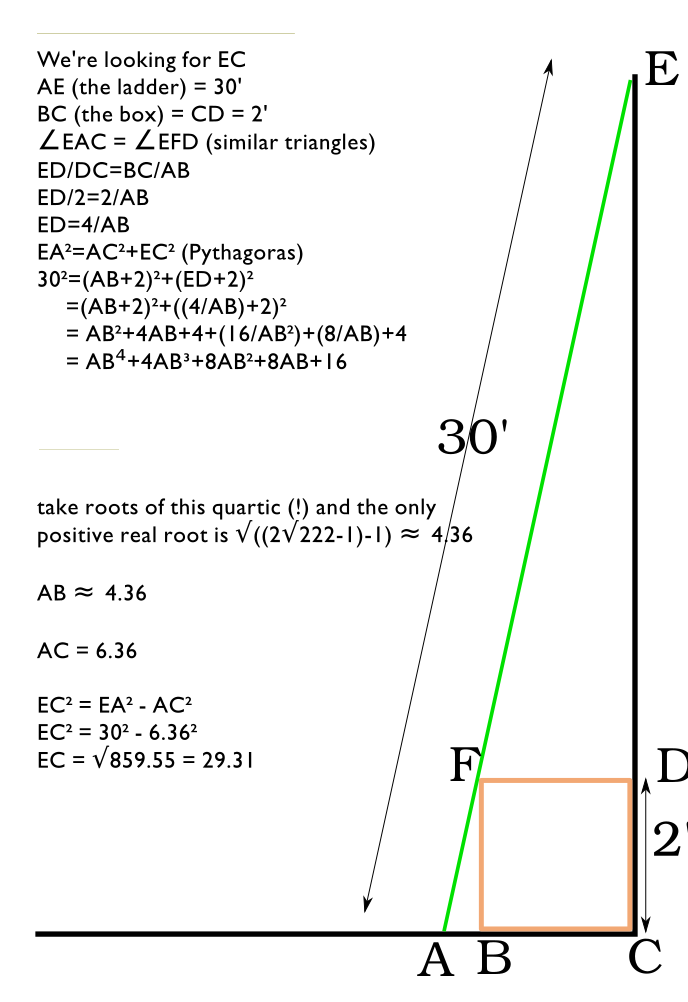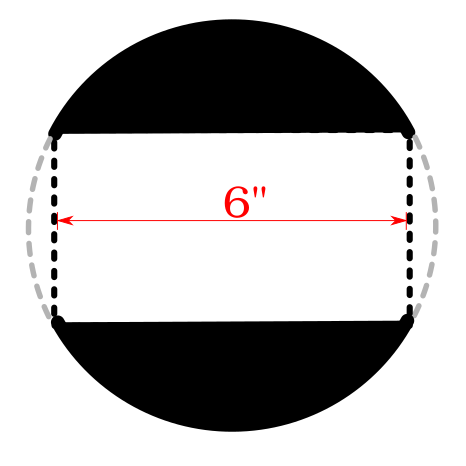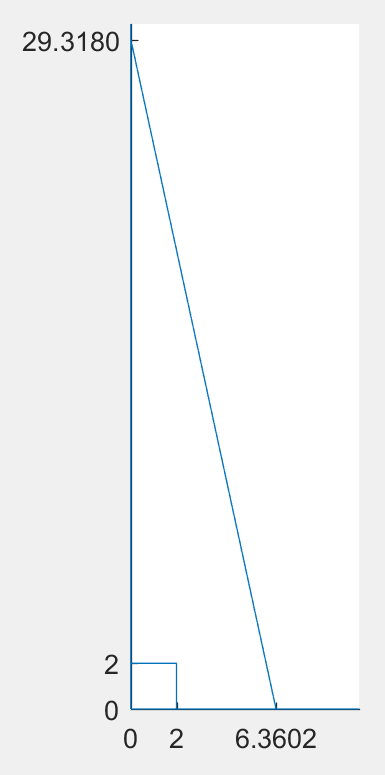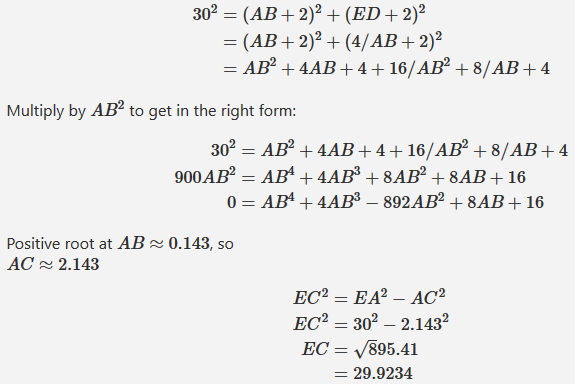# Math(s) To Play With

I work as a computer programmer, well a web developer, PHP FTW. TBH it is mostly a problem domain of lifting data out of a database and making it look nice then pushing it down to a web browser.

I’m keen to broaden my skillset and generally I like to play with things.

So recently I have started looking back at basic Mathematics, long division on paper etc and East Asian algorithms for multiplication.

I’m curious are there any areas of Maths that you guys find particularly interesting and enjoyable to do?

(Lets assume that Boolean algebra and number base’s are given).

Maths is a very interesting subject and is one of the key areas to study. There are several sites catering for mathematical interests, Khan Academy for example.

There are several sites exploring recreational maths, Note being British I recognise this is is a contraction of ‘Mathematics’ and so always plural and never singular.

I’d suggest a structured approach: you will learn more.

## Starter question: In your house you have a 2’ box touching the wall the floor pushed in as tight as it can be. leant against that is 30’ ladder - just touching the floor, the wall and the box. If we assume he height s as big as possible how far do we reach up the wall?

I’m happy to continue this discussion in private (via PM) because I suspect many here are not interested but happy to help you explore your ideas

Tip for my question above if you think about angles this can be reduced to a quadratic equation so solved using the classic equation Otherwise we are looking at a quartic so estimating via Newtons method or similar.

Seriously? A fuckin’ quartic? And you drop this on people at nine at night? That sort of thing ought to be bloody prohibited by the Geneva Convention.

Anyway, here’s my solution. Not sure it’s right; it seems too close to 30 to be real, but I’m not sure where I went wrong, and it’s two o’clock in the frigging morning. And I hope the fleas of a thousand camels infest your armpits for rolling out an easy-looking problem which involves me solving a bastard quartic.1 Like

@nshiell was asking for interesting maths. You did not have to solve this at four in the morning or at all even.

Ha! Very true! I am punished by not being able to let an interesting problem goAnd it was fun to think about; it’s impressive that something which seems so simple is actually a really hard problem. Cheers for giving it – and anyone else who wants to solve it should ignore my solution, which I think might actually be wrong anyway…!

In return, how about another? This one’s a bit old, but it’s good. I have a sphere, and I drill a hole through the middle. The hole is, end-to-end, six inches long. What’s the volume remaining in the sphere?I’m not going to attempt this now but I will give it a go over the weekend and post my answer if I have one and my thoughts on it if I don’t . Do I get to know the diameter of the drill or do I have to work that out too?

Or does it matter? a really big sphere with a large diameter hole or a small one with a tiny hole? Is the answer the same?

@sil please don’t answer these. I’m throwing thoughts out for others to explore.I will post my attempt this weekend.

Is this a cross section of the sphere?

Yup. I didn’t feel up to drawing it in 3dNo need to. I didn’t give it enough thought.

I think I’m lost. The diameter of the hole will, if I understand the drawing and the problem, will affect the diameter of the sphere, which will affect the volume. Right? Example: A 1" hole 6" long will make a sphere of 6.0828" Dia. A 1/2" hole 6" long will make a sphere of 6.0208" Dia. If I have done the math right (BIG IF) then the volume for the sphere with the 1" hole would be 98.994^3 inches and the 1/2" hole would be 113.1^3 inches. (This just can’t be right)

I’m just waiting to give myself a dope slap when the solution is given!1 Like

Approximately 133 square inches

36 pi square inches exactly

1 Like

Does anyone need to see a derivation or proof of my answer?

Happy to provide if wanted.

This is an example of the napkin ring problem. The volume of a sphere with a hole drilled through it is only dependent on the height of the hole drilled. (independent of the radius of the original sphere.)

The formula for a napkin ring is V = pi h^3 / 6 which gives 36pi inches^3 (I converge with @WarrenHill )

Here is a proof

It would be really useful to have LaTeX rendering enabled in this thread.

Fractals are something I find really interesting, but their not exactly solvable, they tend to be infinite and a computer is needed to solve any decent amount of them. So, show me you fractal solving bash scripts! (Actually I’m looking for a good free software fractal renderer. XaoS is all I can find.)

#### The Mandelbrot set

lets define w as some complex number.
lets define the set z as:

z_0 = w, z_1 = z_0^2 + w, z_n = z_(n-1)^2 + w

For what values of w does z converge? An number w where its set z converges to |z| <= 2 is part of the Mandelbrot set.

These points can be rendered black on a plot of the Mandelbrot set, any number w where its set z leaves the area of radius 2 is colour coded by the number of iterations it takes to leave that area. This is what leads to the fractal pattern.You may be familiar with Mathjax, It is used on several stack-exchange sites maths, electronics, physics etc. where people want to display maths that looks like how you would see it in a text book. I understand there is a Discourse plug-in available.

I have not tested it so can’t attest to how good it is. If there is sufficient interest I’d be happy to fire up my own Discourse server to test this. If it looks OK we could run a live test system on my website but this would be dependant on:

1. Sufficient interest to see if it is worth exploring.
2. @sil , @jonobacon and @jeremy being prepared to install it here should the trial be a success. I suspect Stuart would be doing most of the work to implement this: I am not an admin on this site - nor do I want to be; but if we decide to go down this route I’ll be happy to provide all the support I can.

My gut feeling is that given this is the first mathematics thread I can think of there is unlikely to be sufficient interest to justify this. I am however, happy to be proved wrong.

I’m still working out my solution, but there’s an error in yours. I thought the image you’ve drawn looks about right, so an AC distance of 6.36 seems a bit on the large side. Here’s an image of your solution:1 Like

Yeah, I thought I’d done something wrong, but I’m not sure what; I included the working so someone can show me where I screwed upThe root 222 equation doesn’t make sense - the brackets look wrong.

Here you go, simple mistake.Fwiw, I did this in the mathjax preview window. Source is below:

\begin{align}
30^2 & = (AB+2)^2 + (ED+2)^2
\\ & = (AB+2)^2 + (4/AB+2)^2
\\ & = AB^2 + 4AB + 4+16/AB^2 + 8/AB + 4
\end{align}

Multiply by $AB^2$ to get in the right form:

\begin{align}
30^2 & = AB^2 + 4AB + 4+16/AB^2 + 8/AB + 4
\\ 900AB^2 & = AB^4+4AB^3+8AB^2+8AB+16
\\ 0 & = AB^4+4AB^3-892AB^2+8AB+16
\end{align}

Positive root at $AB\approx0.143$, so $\\ AC\approx2.143$

\begin{align}
EC^2 & = EA^2 - AC^2 \\
EC^2 & = 30^2 - 2.143^2 \\
EC & = \sqrt895.41 \\
& = 29.9234
\end{align}
Please respect our code of conduct which is simple: don't be a dick.